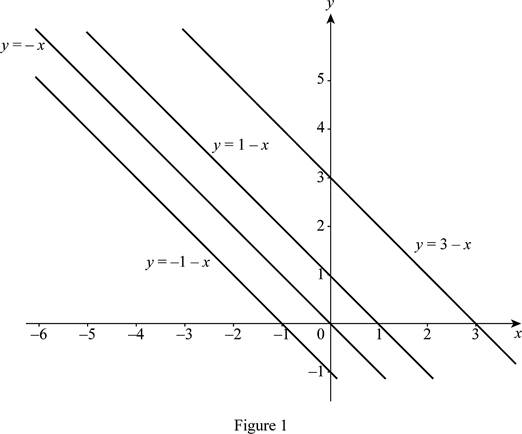# The common term of all the members of the family of linear function f ( x ) = c − x ; sketch the members of the family.### Single Variable Calculus: Concepts...

4th Edition
James Stewart
Publisher: Cengage Learning
ISBN: 9781337687805### Single Variable Calculus: Concepts...

4th Edition
James Stewart
Publisher: Cengage Learning
ISBN: 9781337687805

#### Solutions

Chapter 1.2, Problem 7E
To determine

## To find: The common term of all the members of the family of linear function f(x)=c−x ; sketch the members of the family.

Expert Solution

The members of the family of linear function f(x)=cx have the common slope m=1 and y-intercept is c.

### Explanation of Solution

Consider the function y=cx .

Here, the slope (m) of the function is −1 and y-intercept is c.

Obtain the equations of the function for various c values.

If c=1 , then y=1x .

If c=1 , then y=1x .

If c=0 , then y=x .

If c=3 , then y=3x .

Draw the graph of above equations as shown below in Figure 1.From Figure 1, it is noticed that all the members of the family of linear function y=cx have the common slope m=1 and y-intercept is c.

### Have a homework question?

Subscribe to bartleby learn! Ask subject matter experts 30 homework questions each month. Plus, you’ll have access to millions of step-by-step textbook answers!